Related Articles

# How to Calculate Covariance in MATLAB

• Last Updated : 31 Aug, 2021

Covariance is the measure of the strength of correlation between two or more random variables. Covariance of two random variables X and Y can be defined as:Where E(X) and E(Y) are expectation or mean of random variables X and Y respectively.

The covariance matrix of two random variables A and B is defined asMATLAB language allows users to calculate the covariance of random variables using cov() method. Different syntax of cov() method are:

1. C = cov(A)
2. C = cov(A,B)
3. C = cov(___,w)
4. C = cov(___,nanflag)

## C = cov(A)

• It returns the covariance of array A.
• If A is a scalar, then it returns 0.
• If A is a vector, then it returns the variance of vector A.• If A is a matrix, then it considers each column as a random variable and returns the covariance matrix of matrix A.

Note: disp (x) displays the value of variable X without printing the variable name. Another way to display a variable is to type its name, which displays a leading “X =” before the value. If a variable contains an empty array, disp returns without displaying anything.

Example 1:

## Matlab

 % Input vectorA = [1 3 4];disp("Vector :");disp(A); % Variance of vector AC = cov(A);disp("Variance :");disp(C);

Output :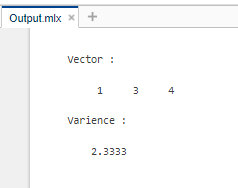Example 2:

## Matlab

 % Input vectorA = [2 7 1;     3 5 1     4 1 2];disp("Matrix :");disp(A); % Covariance of matrix AC = cov(A);disp("Covariance matrix :");disp(C);

Output :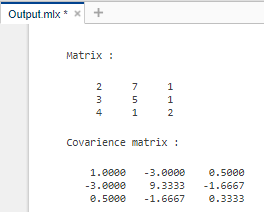## C = cov(A,B)

• It returns the covariance matrix of arrays A and B.
• If A and B vectors, then it returns the covariance matrix of A and B.
• If A and B are matrices, then it considers them as vectors themselves by expanding the dimensions and returns the covariance matrix.

Example:

## Matlab

 % Input vectorA = [3 5 7];B = [-1 3 9];disp("Vector A:");disp(A);disp("Vector B:");disp(B); % Covariance of vectors A,BC = cov(A,B);disp("Covariance matrix :");disp(C);

Output :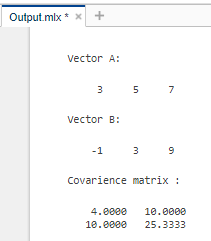## C = cov(___,w)

• It returns the covariance of the input array by normalizing it to w.
• If w = 1, then covariance is normalized by the number of rows in the input matrix.
• If w = 0, then covariance is normalized by the number of rows in the input matrix – 1.

Example:

## Matlab

 % Input vectorA = [2 4 6;     3 5 7     8 10 12];disp("Matrix :");disp(A); % Variance of matrix AC = cov(A,1);disp("Variance matrix:");disp(C);

Output :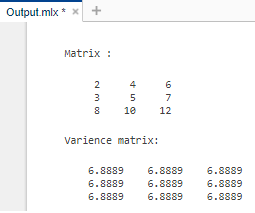## C = cov(___,nanflag)

• It returns the covariance of the input array by considering the nanflag.
• If nanflag = ‘includenan’, then it considers NaN values in array.
• If nanflag = ‘omitrows’, then it omits the rows with at least one NaN value in the array.

Example:

## Matlab

 % Input vectorA = [3.2 -1.005 2.98;     NaN  -6.75  NaN;     5.37  0.19  1]disp("Matrix :");disp(A); % Variance of matrix AC = cov(A,'includenan');disp("Variance matrix including NaN:");disp(C);  % Variance of matrix AC = cov(A,'omitrows');disp("Variance matrix omitting NaN:");disp(C);

Output :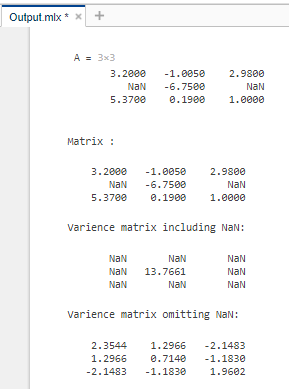My Personal Notes arrow_drop_up